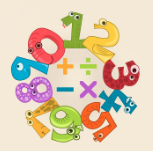# Math Fact Fluency

Empowered Learner### Overview​

Multiplication and division fact fluency is a Common Core grade-level standard for third grade. Students can practice their fact fluency and have some fun at the same time!

They will use the application Fact Monster.

Students will:

• Be able to practice their multiplication and division facts on various levels as they are timed so they can be fluent.

Vocabulary Words:

• Multiply: Multiply is to add a number to itself a particular number of times.

• Divide: Divide is to separate (something) into portions and distribute a share.

• Repeated Addition: Repeated addition is adding equal groups together. It is also known as multiplication. If the same number is repeated then, we can write that in the form of multiplication.

• Repeated Subtraction: Repeated subtraction is the method of subtracting the equal number of items from a larger group.

To prepare for this lesson:

• Assess students for fluency in multiplication and division.

• The teacher should preview the levels on Fact Monster.

• Introduce students to the website http://factmonster.com.

Note: The higher the level that the students go, the more advanced the multiplication, fourth and fifth-grade level. Although this is a simple lesson, many students struggle with math fact fluency.

See Accommodations Page and Charts on the 21things4students.net site in the Teacher Resources.

Directions for this activity:

1. Have the students log onto Factmonster.

2. Students should start at a level that is appropriate.

3. Select level.

4. Click start to begin.

Different options for assessing the students:

• Observations
• Check for understanding
• Assess students with multiplication facts.
• Play a game of around the world multiplication online or with a set of flash cards.

MITECS: Michigan adopted the "ISTE Standards for Students" called MITECS (Michigan Integrated Technology Competencies for Students) in 2018.

Empowered Learner
1a. Students articulate and set personal learning goals, develop strategies leveraging technology to achieve them and reflect on the learning process itself to improve learning outcomes.
1b. Students build networks and customize their learning environments in ways that support the learning process.
1c. Students use technology to seek feedback that informs and improves their practice and to demonstrate their learning in a variety of ways.
1d. Students understand the fundamental concepts of technology operations, demonstrate the ability to choose, use and troubleshoot current technologies and are able to transfer their knowledge to explore emerging technologies.

Browser: Chrome, Safari, Firefox, Edge, ALL